#### You may also like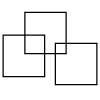### Three Squares

What is the greatest number of squares you can make by overlapping three squares?### Two Dice

Find all the numbers that can be made by adding the dots on two dice.### Biscuit Decorations

Andrew decorated 20 biscuits to take to a party. He lined them up and put icing on every second biscuit and different decorations on other biscuits. How many biscuits weren't decorated?

# Same Length Trains

##### Age 5 to 7 Challenge Level:

We had just a few solutions sent in for this task and here you can see a progression of ideas in the solutions.

Eva from Elmgrove School sent in the following:

I noticed that the white train contained 20 rods. Both of the trains were the same length, but consisted of different numbers of each rod. There were different colours rods. What also caught my eye was that the different colour train's rods were not the same size.

Hebey from Venkateshwar International School in India sent in this image: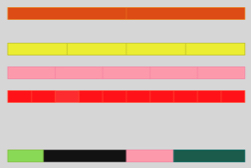Luay from Goldsworth Primary School sent in the following image: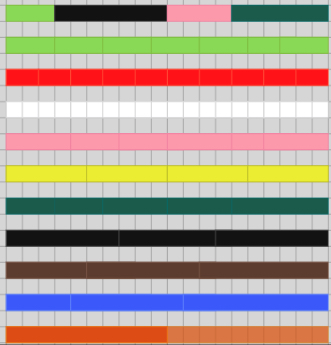Simran from Maurice Hawk School in the USA sent in a Word document as follows:

First, I noticed that if I arrange all the rods from smallest to largest, each rod is 1 unit longer than the previous rod (please see picture).

w= 1 unit, r= 2, g= 3, p= 4, y= 5, d= 6, b= 7, t= 8, B= 9 and o= 10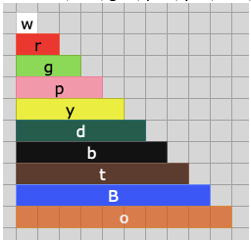The train shown in this picture is made of 20 white rods. To find out which other colour rods can make this train, I decided to skip-count. If you reach 20 using skip-counting, then that colour rod can be used to make the train. The number of times you skip-count tells you how many rods you need to use to make the train.

r (2 units long) = 2, 4, 6, 8, 10, 12, 14, 16, 18, 20 (10 red rods are needed to make the train)

g (3 units long) =3 , 6, 9, 12, 15, 18, 21 (You cannot use the green rod because you don’t get 20 during the skip-count, you can make a train that is 18 units long or 21 units long but not 20 units long)

p (4 units long) = 4, 8, 12, 16, 20 (5 pink rods are needed to make the train)

y (5 units long) = 5, 10, 15, 20 (4 yellow rods are needed to make the train)

d (6 units long) = 6, 12, 18, 24 (Cannot use dark green rods)

b (7 units long) = 7, 14, 21 (Cannot use black rods)

t (8 units long) = 8, 16, 24 (Cannot use tan rods)

B (9 units long) = 9, 18, 27 (Cannot use blue rods)

o (10 units long) = 10, 20 (2 orange rods are needed to make the train)

Red, pink, yellow and orange rods can be used to make the train. When I solved this problem, my brother told me that you can find the factors of 20, which are numbers that can be multiplied to make 20. So, 1, 2, 4, 5 and 10 are factors of 20. By using this strategy, again, red, pink, yellow and orange rods can be used to make the train.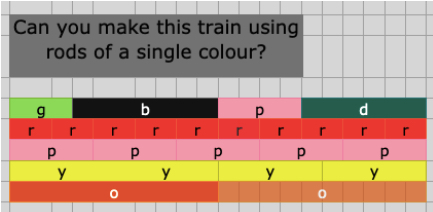Well done to those of you whose work we've seen. We hope to hear from you again in the future!